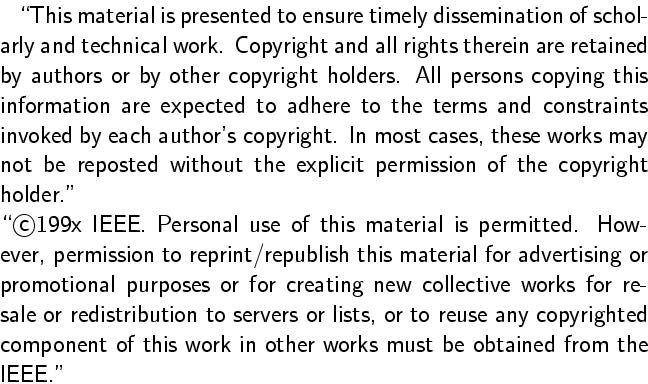Gunther Reißig and Holger Boche.
On singularities of autonomous implicit ordinary differential equations.
IEEE Trans. CAS, Part I, vol. 50, no. 7, July 2003, pp. 922-931.
Full text. (Definitive publication; restricted access.)
Full text. (Free access.)Abstract:
Implicit ordinary differential equations A(x)x'=g(x) with A(x) being an n x n matrix depending on x are investigated about their singular points, i.e., inconsistent points in the closure of the set of consistent points. Sufficient conditions are given that ensure that around such points, the above differential equation can be diffeomorphically transformed into the normal form x1rx1'=+/- 1, x2'=0, ..., xn-m'=0, xn-m+1=0, ...,xn=0 for some r > 0 and some nonnegative m < n. These results are applied to examples from several fields of application, including an electrical network having impasse points.
BibTeX entry:
```@article{ReissigBoche03,
AUTHOR = {Rei{\ss}ig, Gunther and Holger Boche},
TITLE = {On Singularities of Autonomous Implicit Ordinary Differential Equations},
JOURNAL = {IEEE Trans. Circuits Systems I Fund. Theory Appl.},
FJOURNAL = {IEEE Transactions on Circuits and Systems. I. Fundamental Theory and Applications},
VOLUME = {50},
YEAR = {2003},
NUMBER = {7},
PAGES = {922-931},
doi = {10.1109/TCSI.2002.805739}
}
```

Impressum und Haftungsausschluß